# Mrs Perry

Mrs Perry shares out 25 biscuits between Gemma and Zak in the ratio 1:4. How many biscuits has each?

g =  5
z =  20

### Step-by-step explanation:Did you find an error or inaccuracy? Feel free to write us. Thank you!Tips to related online calculators
Need help to calculate sum, simplify or multiply fractions? Try our fraction calculator.
Check out our ratio calculator.

## Related math problems and questions:

• 120 nuts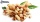Divide 120 nuts in a ratio of 4: 6.
• Ratio 11Simplify this ratio 10 : 1/4
• 4 pipes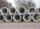The tank flows out by 4 pipes in 6 hours 120 hl water. How much water flows out of 5 pipes of the same diameter in 14 hours?
• Cake ingredients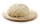The yeast cake contains milk, yeast, sugar, and flour in a ratio of 20: 4: 1: 15. What percentage of flour is in yeast?
• Five numbers in ratioFive integers are in the ratio 1: 2: 3: 4: 5. Their arithmetic mean is 12. Determine the smallest of these numbers.
• The difference 2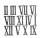The difference between the two numbers is 25. The smaller number is 1/6th of the larger number. What is the value of the smaller number?
• Mrs. Zarka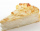Mrs. Zarka has 3 pies for a party. She calculates that if she splits the pies evenly among the guests, they will each receive 1/6 of a pie. How many guests are there?
• Stock market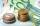Simon one day decided to invest € 62000 to the stock market. After six months, he invested July 25 stock markets fell by 47%. Fortunately for Simon, from July 25 5 to October 25 his shares have risen by 39%. Simon is then: ...
• In the bakery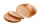In 2.25 hours, 24 pieces of bread are baked in the bakery. How many breads are baked in the bakery in 6 hours and 45 minutes?
• Four classses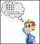Students of all 7, 8 and 9 classes in one school may take up 4,5,6 and 7 abreast and nobody will left. How many is the average count of pupils in one class if there are always four classes each grade?
• Boys and girls 8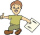In a class of 35 boys and girls that has a ratio of 2:5, 5 boys are absent and 5 boys are present. How many students are present?
• Oranges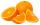Mother divided her three children's oranges in a ratio of 6:5:4. Two children gave 45 oranges. How many oranges were there?
• Four poplarsFour poplars are growing along the way. The distances between them are 35 m, 14 m, and 91 m. At least how many poplars need to be dropped to create the same spacing between the trees? How many meters will it be?
• Dividing moneyMilan divided 360 euros in the ratio 4: 5, Erik in the ratio 500: 625. How many euros did the individual parts of Milan and how many Erik?
• Garden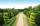The garden has a rectangular shape with lengths of 25 and 40 meters. It has been expanded so that each of its size increased by one fifth. How many square meters increased its acreage?
• Two math problems1) The sum of twice a number and -6 is nine more than the opposite of that number. Find the number. 2) A collection of 27 coins, all nickels, and dimes, is worth \$2.10. How many of each coin are there? The dime, in United States usage, is a ten-cent coin.
• Twenty-fiveHow many are three-digit natural numbers divisible by 25?